# Inference for the mean vector Univariate Inference Let

• Slides: 63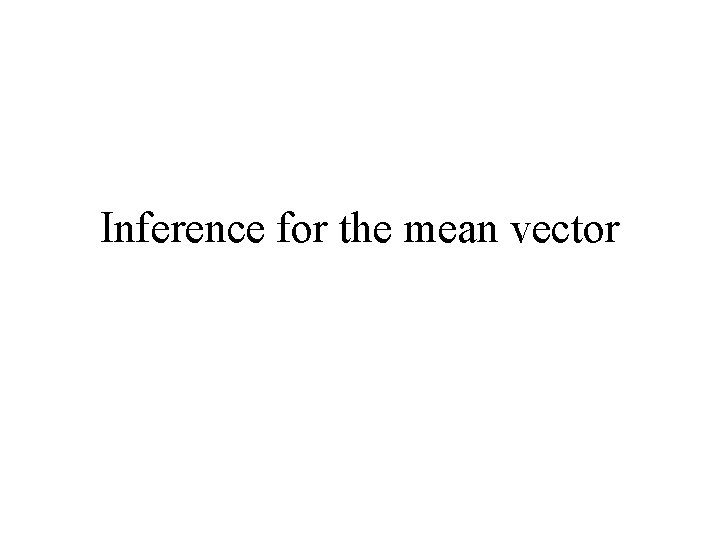Inference for the mean vectorUnivariate Inference Let x 1, x 2, … , xn denote a sample of n from the normal distribution with mean m and variance s 2. Suppose we want to test H 0: m = m 0 vs HA : m ≠ m 0 The appropriate test is the t test: The test statistic: Reject H 0 if |t| > ta/2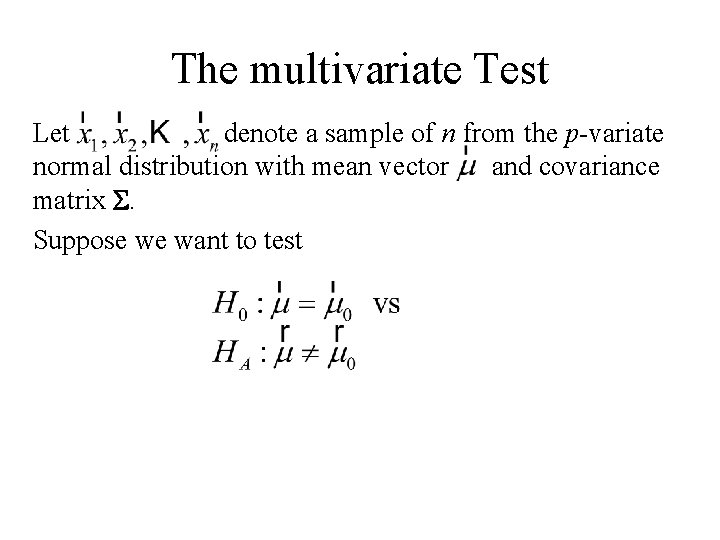The multivariate Test Let denote a sample of n from the p-variate normal distribution with mean vector and covariance matrix S. Suppose we want to testRoy’s Union- Intersection Principle This is a general procedure for developing a multivariate test from the corresponding univariate test. 1. Convert the multivariate problem to a univariate problem by considering an arbitrary linear combination of the observation vector.2. 3. 4. 5. 6. Perform the test for the arbitrary linear combination of the observation vector. Repeat this for all possible choices of Reject the multivariate hypothesis if H 0 is rejected for any one of the choices for Accept the multivariate hypothesis if H 0 is accepted for all of the choices for Set the type I error rate for the individual tests so that the type I error rate for the multivariate test is a.Application of Roy’s principle to the following situation Let denote a sample of n from the p-variate normal distribution with mean vector and covariance matrix S. Suppose we want to test Then u 1, …. un is a sample of n from the normal distribution with mean and variance.to test we would use the test statistic:and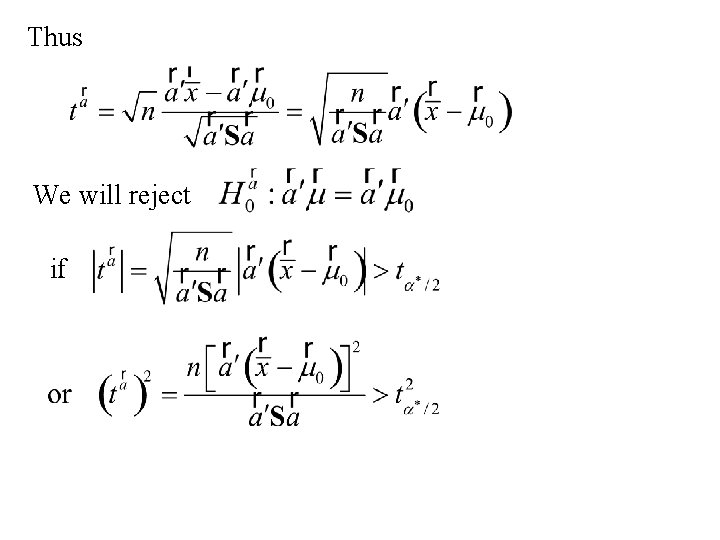Thus We will reject ifUsing Roy’s Union- Intersection principle: We will reject We accept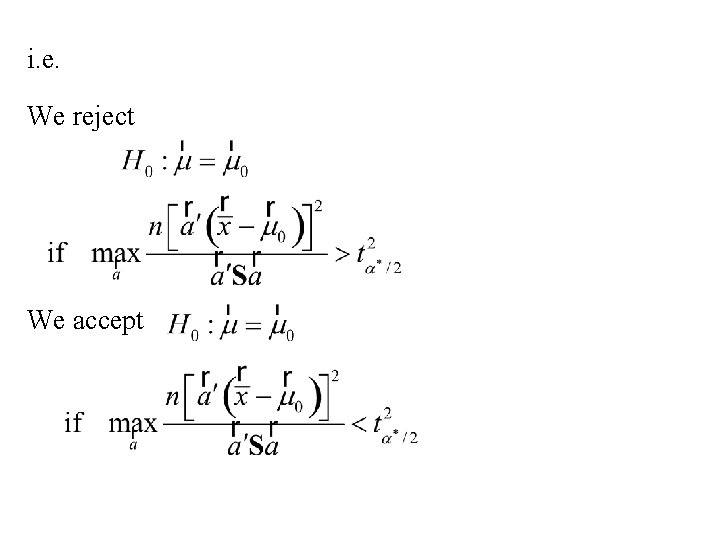i. e. We reject We acceptConsider the problem of finding: wherethusThus Roy’s Union- Intersection principle states: We reject We accept is called Hotelling’s T 2 statisticChoosing the critical value for Hotelling’s T 2 statistic We reject , we need to find the sampling distribution of T 2 when H 0 is true. It turns out that if H 0 is true than has an F distribution with n 1 = p and n 2 = n - p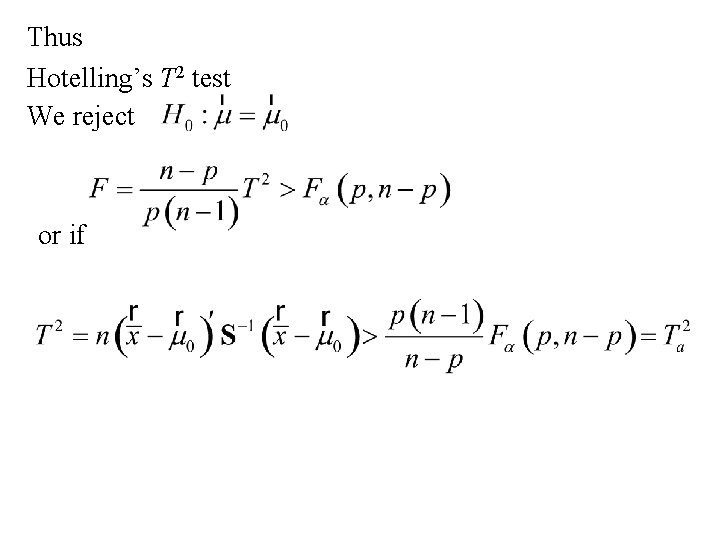Thus Hotelling’s T 2 test We reject or ifAnother derivation of Hotelling’s T 2 statistic Another method of developing statistical tests is the Likelihood ratio method. Suppose that the data vector, , has joint density Suppose that the parameter vector, , belongs to the set W. Let w denote a subset of W. Finally we want to test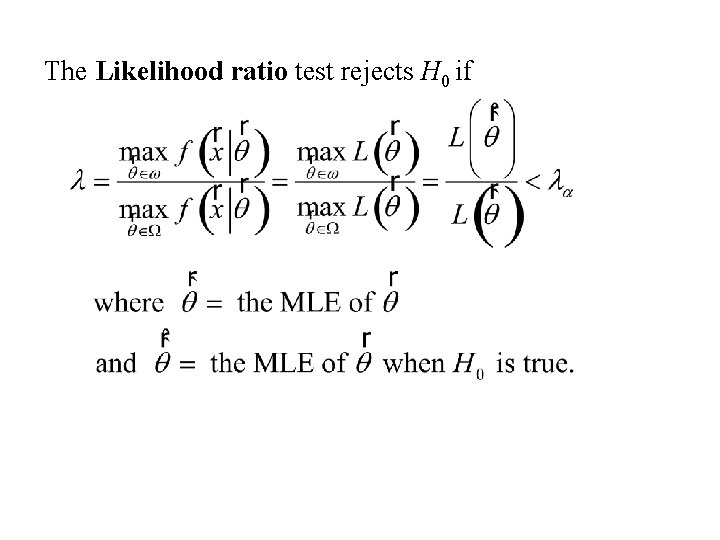The Likelihood ratio test rejects H 0 ifThe situation Let denote a sample of n from the p-variate normal distribution with mean vector and covariance matrix S. Suppose we want to testThe Likelihood function is: and the Log-likelihood function is: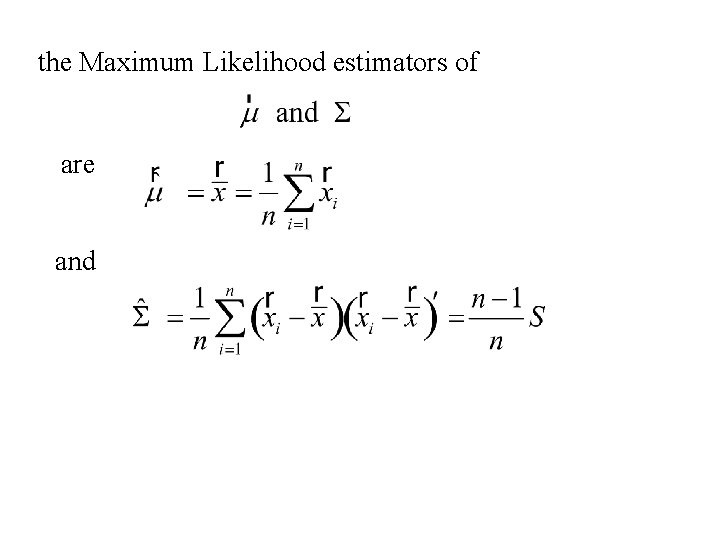the Maximum Likelihood estimators of are and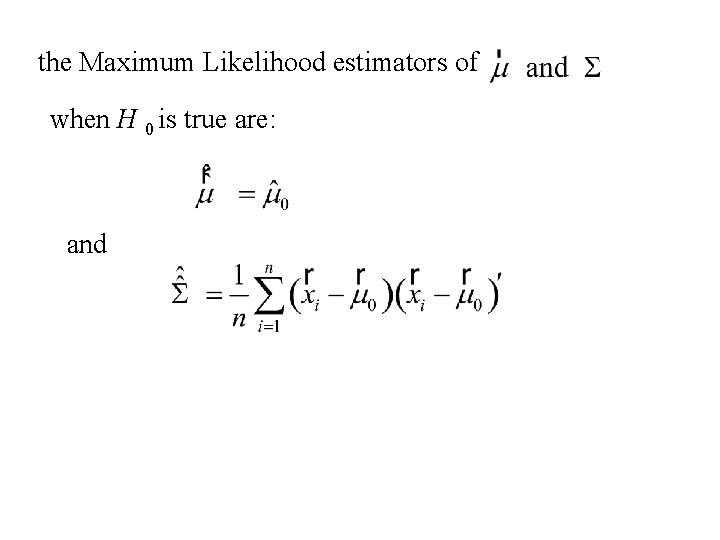the Maximum Likelihood estimators of when H 0 is true are: andThe Likelihood function is: nowThus similarlyandNote: Letand Now andAlsoThusThus using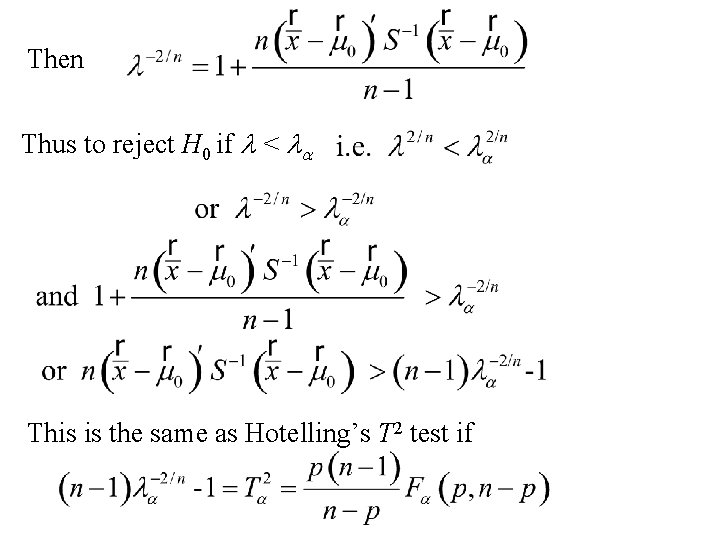Then Thus to reject H 0 if l < la This is the same as Hotelling’s T 2 test if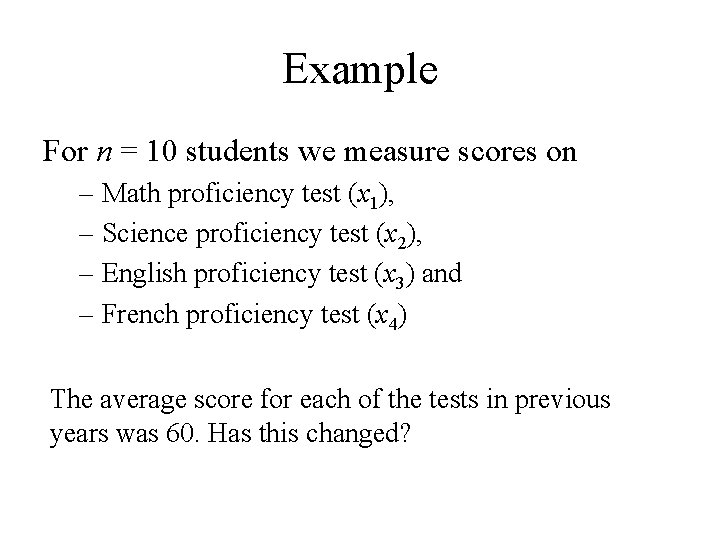Example For n = 10 students we measure scores on – Math proficiency test (x 1), – Science proficiency test (x 2), – English proficiency test (x 3) and – French proficiency test (x 4) The average score for each of the tests in previous years was 60. Has this changed?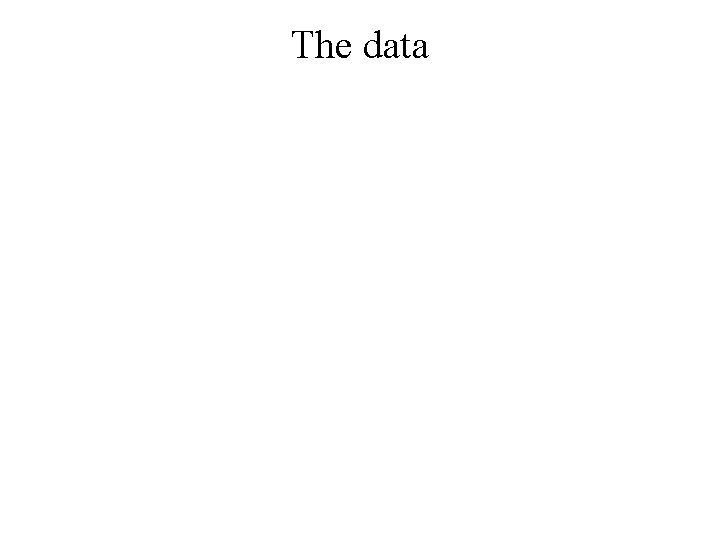The data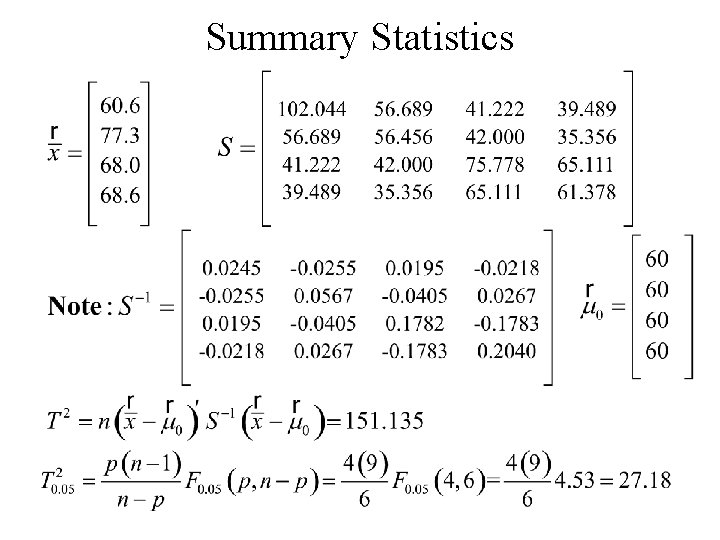Summary StatisticsSimultaneous Inference for means Recall (Using Roy’s Union Intersection Principle)NowThus and the set of intervals Form a set of (1 – a)100 % simultaneous confidence intervals forRecall Thus the set of (1 – a)100 % simultaneous confidence intervals for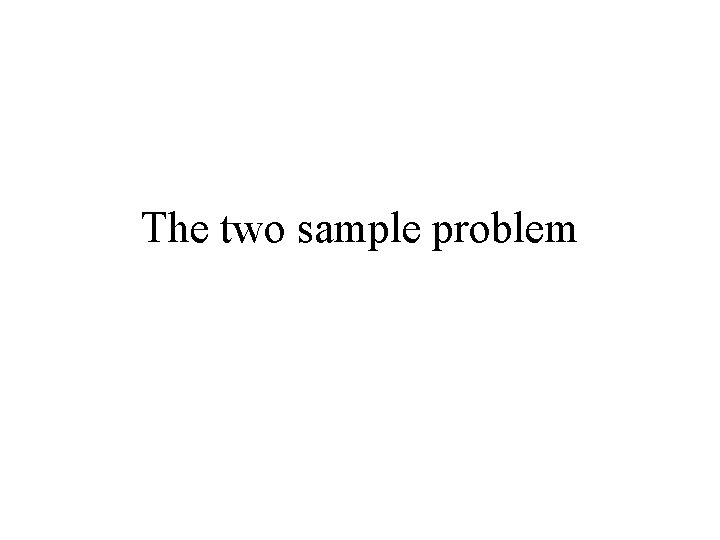The two sample problem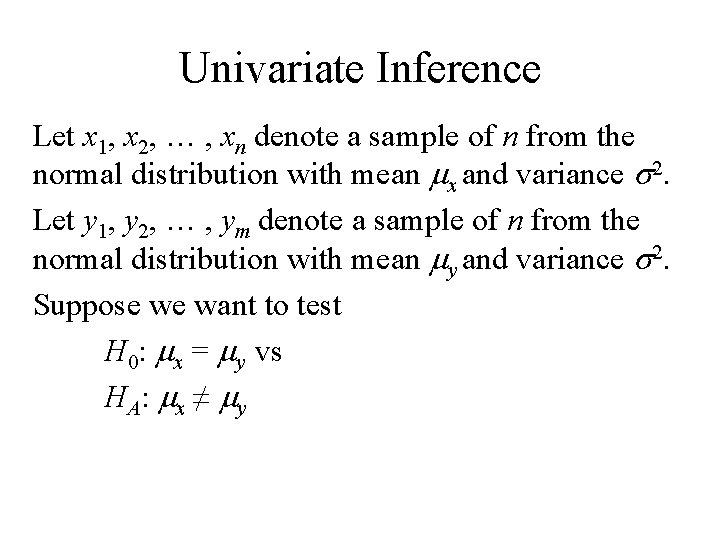Univariate Inference Let x 1, x 2, … , xn denote a sample of n from the normal distribution with mean mx and variance s 2. Let y 1, y 2, … , ym denote a sample of n from the normal distribution with mean my and variance s 2. Suppose we want to test H 0: mx = my vs HA : mx ≠ myThe appropriate test is the t test: The test statistic: Reject H 0 if |t| > ta/2 d. f. = n + m -2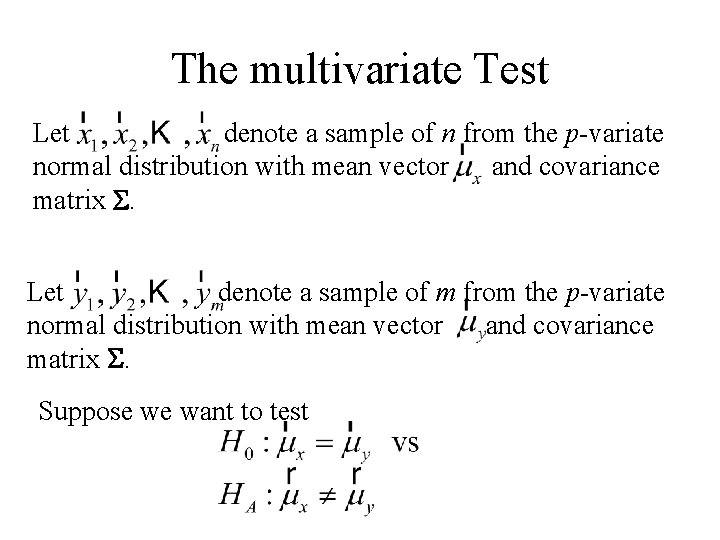The multivariate Test Let denote a sample of n from the p-variate normal distribution with mean vector and covariance matrix S. Let denote a sample of m from the p-variate normal distribution with mean vector and covariance matrix S. Suppose we want to test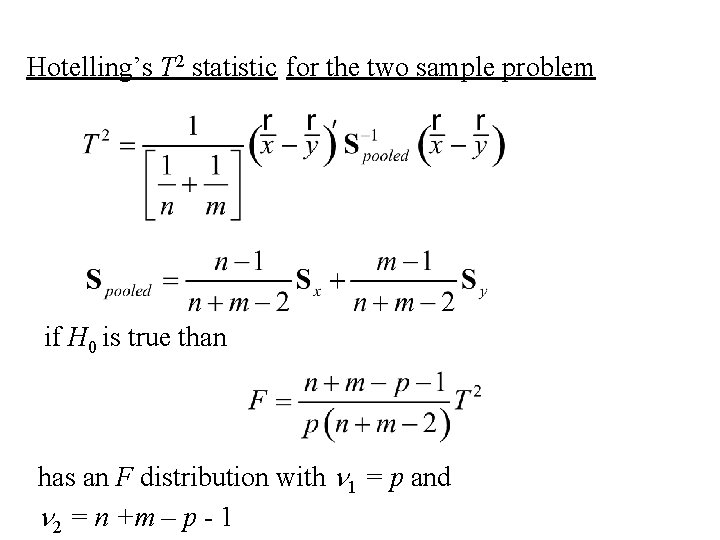Hotelling’s T 2 statistic for the two sample problem if H 0 is true than has an F distribution with n 1 = p and n 2 = n +m – p - 1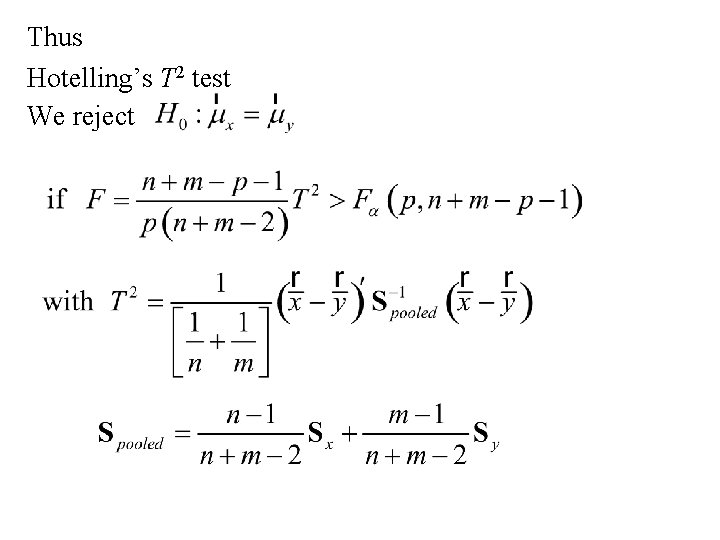Thus Hotelling’s T 2 test We rejectSimultaneous inference for the two-sample problem • Hotelling’s T 2 statistic can be shown to have been derived by Roy’s Union-Intersection principleThus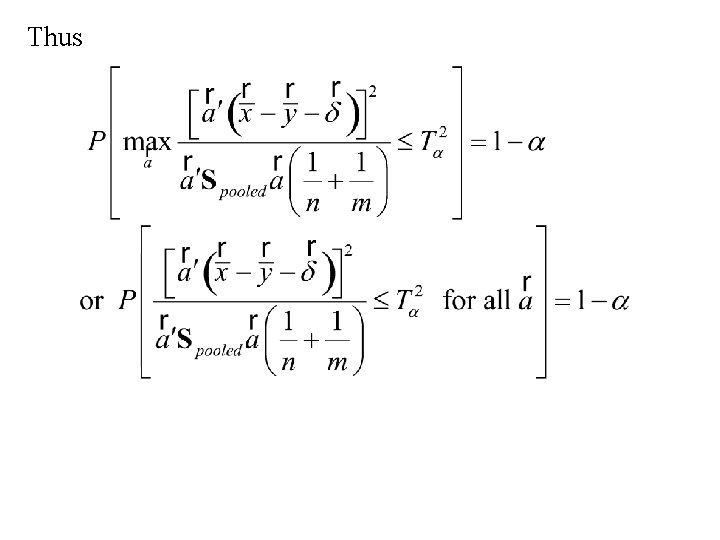Thus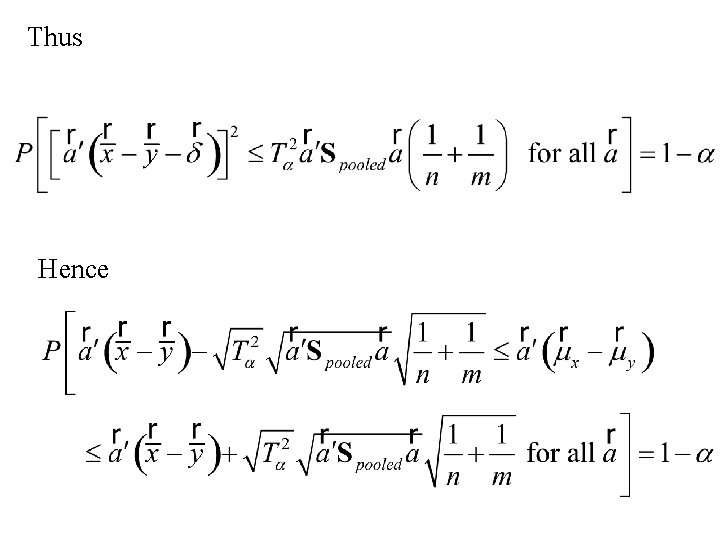Thus Hence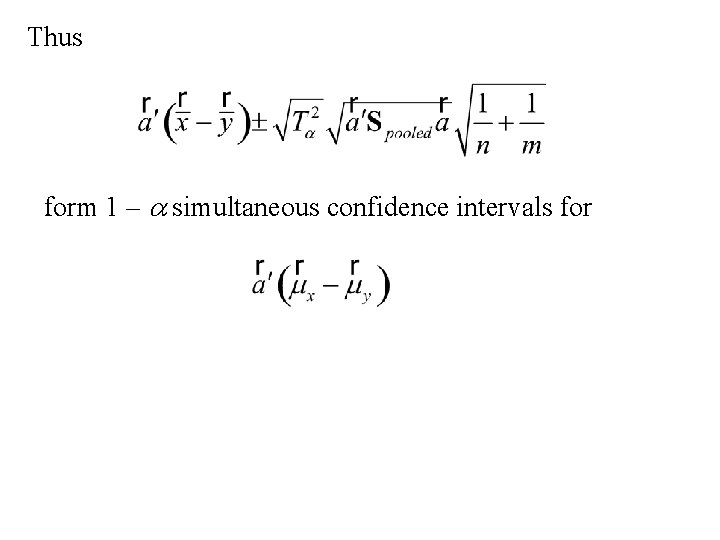Thus form 1 – a simultaneous confidence intervals forHotelling’s T 2 test A graphical explanationHotelling’s T 2 statistic for the two sample problemis the test statistic for testing:Hotelling’s T 2 test X 2 Popn A Popn B X 1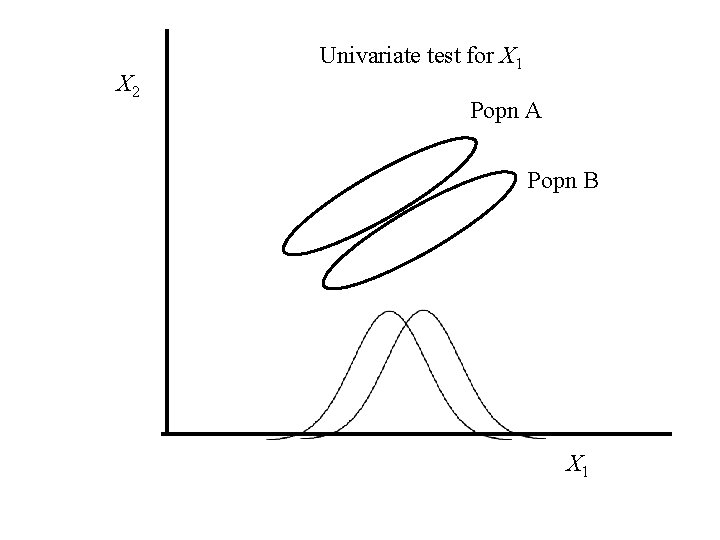X 2 Univariate test for X 1 Popn A Popn B X 1X 2 Univariate test for X 2 Popn A Popn B X 1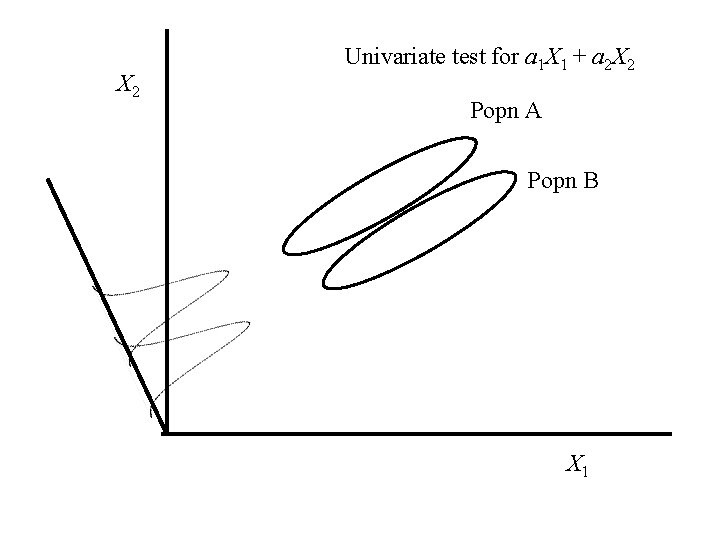X 2 Univariate test for a 1 X 1 + a 2 X 2 Popn A Popn B X 1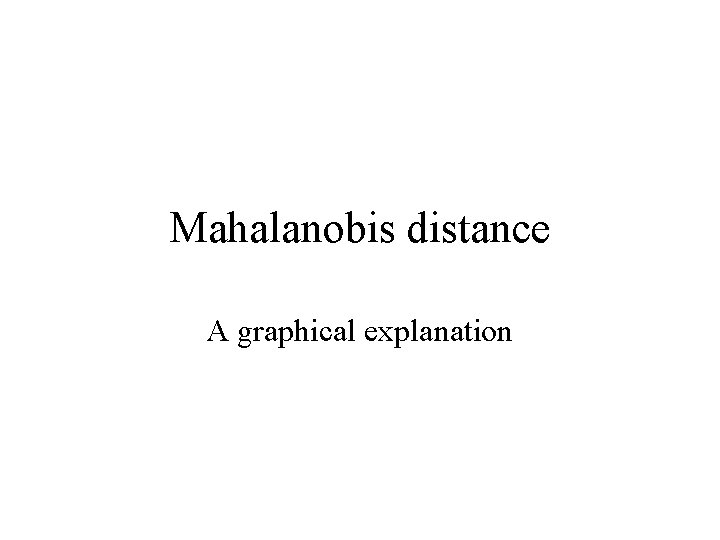Mahalanobis distance A graphical explanationEuclidean distance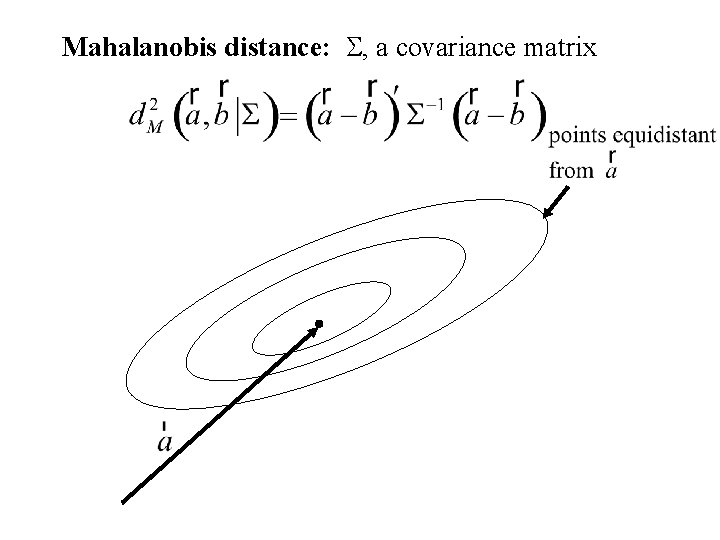Mahalanobis distance: S, a covariance matrix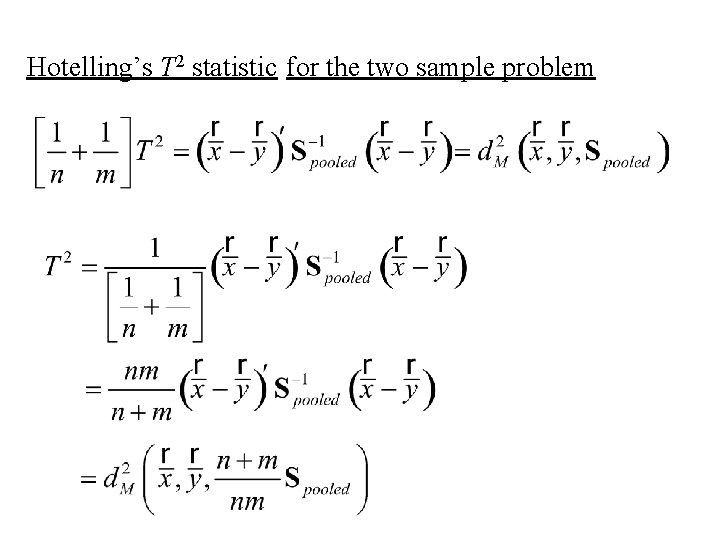Hotelling’s T 2 statistic for the two sample problem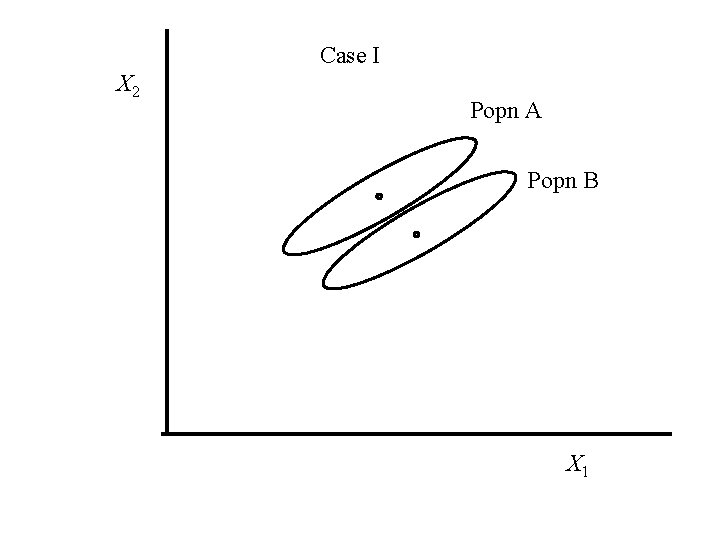Case I X 2 Popn A Popn B X 1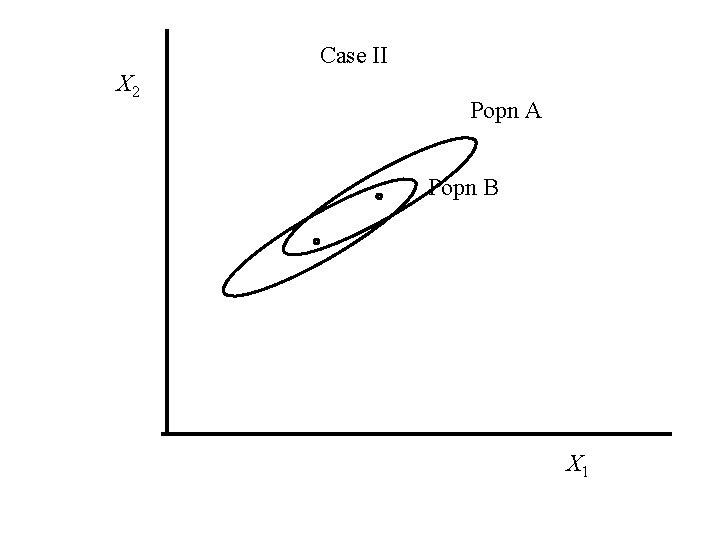Case II X 2 Popn A Popn B X 1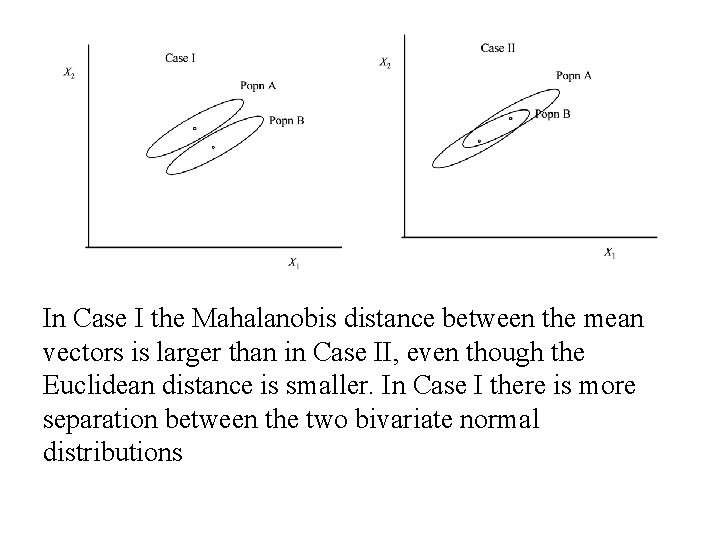In Case I the Mahalanobis distance between the mean vectors is larger than in Case II, even though the Euclidean distance is smaller. In Case I there is more separation between the two bivariate normal distributions